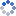加载中…氯化钡和硫酸银
• 博客等级：• 博客积分：0
• 博客访问：91,145
• 关注人气：37
• 获赠金笔：0支
• 赠出金笔：0支
• 荣誉徽章：

## 数论学习笔记（12）：Pell 方程解的存在性及通解公式(2014-06-07 18:59:08)

### 不等式估计

具有如下形式的不定方程称为 Pell 方程：其中 d 为正整数且不是完全平方数。这篇文章只证明一个定理：

设 d 为正整数且不是完全平方数，则方程 x^2-dy^2=1 总有正整数解，且若最小正整数解为 (x_1,y_1) ，则所有正整数解 (x_n,y_n) 满足其中 n=1,2,3,4,...

在证明之前先简单看一下 d 为完全平方数的情况。若 d=k^2 ，则左边可以因式分解为 (x+ky)(x-ky) ，这两个数要么同为 1 要么同为 -1 。解得两组整数解 (x,y)=(1,0),(-1,0) 。

当 d 不是完全平方数时，显然有 x 不为 0 ，而若 y=0 则有两组整数解 (1,0),(-1,0) ，剩下 x,y 均不为 0 的情况，我们可以不妨设 x,y 为正整数。

先证明方程有正整数解。第一步是把左边因式分解：我们希望 x-y√d 尽可能小（这样才能期望两个数的乘积为 1 ），为此先证明一个定理：

不等式有无穷多组正整数解。

如果 x,y 满足这个不等式，则 x-y√d 的值可以认为是比较小的。这个定理证明起来并不难，我们用抽屉原理就可以证明它。

事实上，我们要做的事就是找到尽可能接近整数的 y√d 。我们任取正整数 y_0 并寻找不等式满足 y 不超过 y_0 的解 (x,y) ，这样不等式右边是跟 x,y 无关的值，解起来更容易。考虑 y_0+1 个数它们的小数部分都是大于等于 0 小于 1 的实数。（对于任意实数 a ，定义 a 的整数部分为最大的不超过 a 的整数，记作 [a] ，小数部分 {a} 定义为 {a}=a-[a] ）现在造 y_0 个区间作为抽屉，然后把刚才那 y_0+1 个数按照小数部分放到 y_0 个抽屉中，必然有一个抽屉至少含有两个数，这两个数小数部分的差的绝对值显然小于 1/y_0 ，设这两个数分别为 u√d,v√d ，不妨设 u>v 。于是有注意到 u-v 必然不超过 y_0 ，于是找到了不等式 |x-y√d|<1/y_0 的一组解容易证明 x,y 均为正整数（因为 u√d-v√d 必定大于 1 ，所以 x 不可能等于 0 ）。

注意到不等式 |x-y√d|<1/y_0 满足 y<=y_0 的正整数解 (x,y) 必然也满足不等式 |x-y√d|<1/y （因为显然有 1/y_0<=1/y ）。于是我们让 y_0 取遍所有正整数就可以得到无穷组 |x-y√d|<1/y 的正整数解。

最后一点，要是这些解重复了怎么办？重复解当然有可能出现，不过我们可以证明有无穷组不同的正整数解：我们让 y_0 从 1 开始不断增大，则不会有一个解重复无限多次（假如有这样的解 (x,y) ，则 |x-y√d| 一定是个正数，随着 y_0 的增加，不等式 |x-y√d|<1/y_0 从某一刻开始永远不成立，这样这个解最多在这一刻之前重复有限多次，矛盾）。

随着 y_0 从 1 开始不断增大，总会有新的解出现。这就产生了无穷组不同的 |x-y√d|<1/y 的正整数解。

至此我们就证得了这个定理，事实上 √d 可以换为任意正无理数，证明是一样的。

接下来开始证明 Pell 方程定理的第一部分：方程 x^2-dy^2=1 必有正整数解。为此先随便取一组不等式 |x-y√d|<1/y 的正整数解 (x,y) 估计一下 x^2-dy^2 的值：放缩的幅度比较大，不过这个无所谓，关键是最后的上界 3√d 和下界 -3√d 都是与 x,y 无关的常数，这告诉我们 x^2-dy^2 作为一个整数只有有限个可能的取值。

我们已经证过这样的 x,y 有无穷组，再次使用抽屉原理，我们总能挑出两组解 (x_1,y_1),(x_2,y_2) 使得接下来是关键，我们让两式相除，当然为了容易得到结果，我们用 x_1+y_1√d 除以 x_2+y_2√d ：于是我们猜到了 x^2-dy^2=1 的一组解为经验证它确实满足 x^2-dy^2=1 。不过很不幸的是，它不一定是整数解。我们要想办法补救它，注意到当时，这组解必为整数解。再回头看，事实上我们只挑出两组解 (x_1,y_1),(x_2,y_2) 太少了，我们可以直接挑出来无穷多组解 (x_i,y_i) 都满足方程 x^2-dy^2=k （注意我们是把无穷多组解放到有限多个抽屉里）。

然后，从这无穷多组解里再挑两组模 k 同余的解，这太简单了，仍然是抽屉原理，我们要把无穷多组满足 x^2-dy^2=k 的解按照除以 |k| 的余数放到 |k|^2 个抽屉 (i,j) 中（其中 i,j=0,1,2,3,...,|k|-1 ），必然有一个抽屉含有至少两组解。

注意 k 显然不能为 0 ，否则 √d=x/y 为有理数矛盾。

把这两组解记为 (x_1,y_1),(x_2,y_2) ，这时解便是一组整数解。

最后验证它的确是正整数解，首先这显然是一组非负整数解，其次既然它满足 x^2-dy^2=1 那么 x 必然不为 0 ，最后若 y=0 ，则容易得到这与 (x_1,y_1),(x_2,y_2) 是不同的解矛盾，因此 y 不为 0 。

至此，我们已经证明了方程 x^2-dy^2=1 存在正整数解。

接下来证明 Pell 方程定理的第二部分。假设方程 x^2-dy^2=1 有一组大于最小解 (x_1,y_1) 的正整数解 (x,y) （这里的“大于”指 x>x_1 且 y>y_1 ，显然其中一个成立则另一个也成立）。我们期待下式能够给出比 (x,y) 更小的一组正整数解 (x',y') （这里“小于”含义与上述“大于”类似），这样我们也许可以用递降法推出最终结论。把这个式子的右边展开，再对照系数得到关于 x',y' 的方程组然后把这个方程组解出来，结果是显然两个分母都等于 1 ，因此实际上就是显然 x',y' 都是整数，经验证 (x',y') 的确是 x^2-dy^2=1 的一组解。接下来证明 (x',y') 是一组正整数解。先证明 x'>0 ：再证明 y'>0这样就证明了 (x',y') 是方程 x^2-dy^2=1 的一组正整数解。

最后证明 (x',y') 小于 (x,y) ，这个好证，由式子

显然（注意这两个式子里所有变量都是正整数）

接下来使用递降法：设 (x,y) 是方程 x^2-dy^2=1 的一组正整数解，如果它不是最小解 (x_1,y_1) （因此它一定比最小解大），那么用上述方法算出来 (x',y') 去替换 (x,y) ，得到一组更小正整数解。重复此操作，当然操作不能一直进行下去（因为正整数不能无限变小），于是操作必然因为得到解 (x_1,y_1) 而终止。

设这种操作进行了 n 次（ n 可以为任意正整数，这样把 (x,y) 本身是 (x_1,y_1) 的情况考虑了进去），那么由重复代入 n 次就得到其中 n+1 为任意正整数，用 n 替换 n+1 我们就证到了 Pell 方程定理的第二部分。

至此开始提出的 Pell 方程定理已经证完。

0